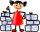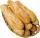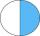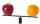Fractions

Sort fractionsby its size. Result write as three serial numbers 1,2,3.

Result

n(z1):  2
n(z2):  1
n(z3):  3

Solution:Leave us a comment of example and its solution (i.e. if it is still somewhat unclear...):Be the first to comment!To solve this example are needed these knowledge from mathematics:

Do you want to calculate least common multiple two or more numbers? Need help calculate sum, simplify or multiply fractions? Try our fraction calculator.

Next similar examples:

1. Comparing and sortingArrange in descending order this fractions: 2/7, 7/10 & 1/2
2. Fraction and a decimalWrite as a fraction and a decimal. One and two plus three and five hundredths
3. Paper collectingAt the paper collecting contest gathered Franta 2/9 ton, Karel 1/4 ton and Patrick 19/36 tons of paper. Who have gathered the most and the least (x, y - write down the weights in tonnes).
4. Mixed2improperWrite the mixed number as an improper fraction. 166 2/3
5. ZdeněkZdeněk picked up 15 l of water from a 100-liter full-water barrel. Write a fraction of what part of Zdeněk's water he picked.
6. Fraction to decimalWrite the fraction 3/22 as a decimal.
7. BuingBrother got to buy 240 CZK and could buy for 1/8 what he wanted. Could he pay the rest of the purchase for 200 CZK?
8. RollsMom bought 13 rolls. Dad ate 3.5 rolls. How many rolls left when Peter yet put two at dinner?
9. Reduce to lowest termsReduce to lowest terms : 32/124
10. DenominatorCalculate the missing denominator x: ?
11. CakesOn the bowl were a few cakes. Jane ate one-third of them, Dana ate a quarter of those cakes that remained. a) What part (of the original number of cakes) Dana ate? b) At least how many cakes could be (initially) on thebowl?
12. Lengths of the poolMiguel swam 6 lengths of the pool. Mat swam 3 times as far as Miguel. Lionel swam 1/3 as far as Miguel. How many lengths did mat swim?
13. In fractionsAn ant climbs 2/5 of the pole on the first hour and climbs 1/4 of the pole on the next hour. What part of the pole does the ant climb in two hours?Added together and write as decimal number: LXVII + MLXIVCommon multiple of three numbers is 3276. One number is in this number 63 times, second 7 times, third 9 times. What are the numbers?What is the smallest number that can be divided by both 5 and 7Compare with characters >, <, =: 85.57 ? 80.83